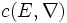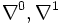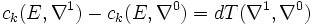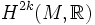# Chern-Weil theorem

Let$c$ denote the Chern form, viz$c(E,\nabla)$ is the Chern form for vector bundle$E$ and connection$\nabla$ on$E$. Then:
•$c_k(E,\nabla)$ are closed for all$k$ and all$\nabla$
• For all positive integers$k$, there exists a$(2k-1)$ form$T$ such that for all$\nabla^0,\nabla^1$ on$E$, we have:$c_k(E,\nabla^1) - c_k(E,\nabla^0) = dT(\nabla^1,\nabla^0)$
Thus,$c_k$ defines an element in$H^{2k}(M,\R)$ independent of$\nabla$. This elements is termed the$k^{th}$ Chern class (the word class because it is a cohomology class).